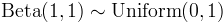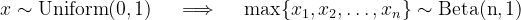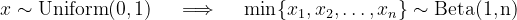Represents a beta distribution.Inheritance Hierarchy

Namespace:  Meta.Numerics.Statistics.Distributions
Assembly:  Meta.Numerics (in Meta.Numerics.dll) Version: 4.1.4Syntax
`public sealed class BetaDistribution : ContinuousDistribution`

The BetaDistribution type exposes the following members.Constructors
NameDescriptionBetaDistribution
Initializes a new β distribution.
TopProperties
NameDescriptionAlpha
Gets the left shape parameter.Beta
Gets the right shape parameter.ExcessKurtosis
Gets the excess kurtosis of the distribution.
(Inherited from UnivariateDistribution.)Mean
Gets the mean of the distribution.
(Overrides UnivariateDistributionMean.)Median
Gets the median of the distribution.
(Inherited from ContinuousDistribution.)Skewness
Gets the skewness of the distribution.
(Overrides UnivariateDistributionSkewness.)StandardDeviation
Gets the standard deviation of the distribution.
(Inherited from UnivariateDistribution.)Support
Gets the interval over which the distribution is non-vanishing.
(Overrides ContinuousDistributionSupport.)Variance
Gets the variance of the distribution.
(Overrides UnivariateDistributionVariance.)
TopMethods
NameDescriptionCentralMoment
Computes a central moment of the distribution.
(Overrides ContinuousDistributionCentralMoment(Int32).)Cumulant
Computes a cumulant of the distribution.
(Inherited from UnivariateDistribution.)Equals
Determines whether the specified object is equal to the current object.
(Inherited from Object.)ExpectationValue
Computes the expectation value of the given function.
(Inherited from ContinuousDistribution.)FitToSample
Computes the Beta distribution that best fits the given sample.GetHashCode
Serves as the default hash function.
(Inherited from Object.)GetRandomValue
Generates a random variate.
(Overrides ContinuousDistributionGetRandomValue(Random).)GetRandomValues
Generates the given number of random variates.
(Inherited from ContinuousDistribution.)GetType
Gets the Type of the current instance.
(Inherited from Object.)Hazard
Computes the hazard function.
(Inherited from ContinuousDistribution.)InverseLeftProbability
Returns the point at which the cumulative distribution function attains a given value.
(Overrides ContinuousDistributionInverseLeftProbability(Double).)InverseRightProbability
Returns the point at which the right probability function attains the given value.
(Overrides ContinuousDistributionInverseRightProbability(Double).)LeftProbability
Returns the cumulative probability to the left of (below) the given point.
(Overrides ContinuousDistributionLeftProbability(Double).)ProbabilityDensity
Returns the probability density at the given point.
(Overrides ContinuousDistributionProbabilityDensity(Double).)RawMoment
Computes a raw moment of the distribution.
(Overrides ContinuousDistributionRawMoment(Int32).)RightProbability
Returns the cumulative probability to the right of (above) the given point.
(Overrides ContinuousDistributionRightProbability(Double).)ToString
Returns a string that represents the current object.
(Inherited from Object.)
TopRemarks

The beta distribution is defined on the interval [0,1]. Depending on its two shape parameters, it can take on a variety of forms on this interval.

The left shape parameter α controls the shape of the distribution near the left endpoint x = 0. The right shapre paramater β controls the shape of the distribution near the right endpoint x = 1. If a shape parameter is less than one, the distribution is singular on that side. If a shape parameter is greater than one, the distribution does to zero on that side. If a shape parameter is equal to one, the distribution goes to a constant on that side.

If the two shape parameters are equal, the distribution is symmetric.

When both shape parameters are one, the beta distribution reduces to a standard uniform distribution.Beta distributions describe the maximum and minimum values obtained from multiple, independent draws from a standard uniform distribution. For n draws, the maximum value is distributed as B(n,1).Similarly, the minimum value is distributed as B(1,n).In fact, the ith order statistic (ith smallest value) in n draws from a uniform distribution is distributed as B(i, n - i + 1).

Because of the wide variety of shapes it can take, the beta distribution is sometimes used as an ad hoc model to fit distributions observed on a finite interval.See Also